ISEE Middle Level Math : Data Analysis

Example Questions

Example Question #61 : Data Analysis And Probability

What is the range of the set below?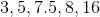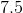Explanation:

The range of a set is the difference between the largest number and the smallest number in the set. Given that 16 is the largest number and 3 is the smallest, the range is equal to 16 minus 3. The result is 13, which is the correct answer.

Example Question #61 : Data Analysis

Find the range of the following set of numbers: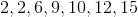Explanation:

To solve, simply find the difference between the smallest and the largest number.In this particular case the largest number is 15 and the smallest number is 2.

Thus,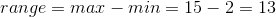Example Question #61 : Data Analysis

If the maximum value in a set isand the range of the set is, then what would the minimum value be?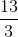Explanation:

The range of a set is the maximum minus the minimum.  We know the maximum isand the range isso you can subtract the range from the maximum value to find you minimum value.  The minimum would then be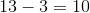.

Example Question #61 : Range

How would the range of a set change if ALL the values are decreased by?

Decreases

Cannot be determined

Increases

Stays the same

Stays the same

Explanation:

The range of a set is the difference between the largest value and the smallest value.  If both values are changed by the same amount, then the difference between will not change and thus the range is the same.

Example Question #63 : Data Analysis

Find the range of the following data set: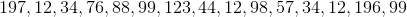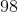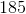Explanation:

Find the mode of the following data set:First, let's rearrange our numbers in increasing order: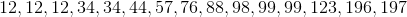Next, identify the range by subtracting the first number from the last number: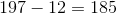So our range is 185

Example Question #64 : Data Analysis

Use the following set to answer the question.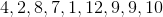Find the range.Explanation:

To find the range of a data set, you find the difference of the smallest and largest number within the set.  So, given the setthe smallest number is 1 and the largest number is 12.  The difference of those two numbers is 11.

Therefore, the range of the data set is.

Example Question #62 : Data Analysis And Probability

Find the range of the following data set: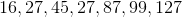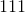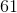Explanation:

Find the range of the following data set:To find the range, simply find the difference between the largest and smallest terms in our series: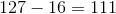So our range is 111

Example Question #65 : Data Analysis

Use the following data set to answer the question: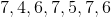Find the range.Explanation:

To find the range of a data set, we will find the difference of the smallest and largest number within the set.  So, given the setwe can see the smallest number isand the largest number is.  To find the difference of these two numbers, we will subtract them.  So,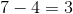Therefore, the range of the data set is.

Example Question #67 : Range

Find the range in this set of numbers: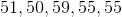Explanation:

To solve, first order the numbers from least to greatest: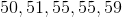Then, subtract the smallest number from the largest: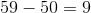Answer: The range isExample Question #62 : Data Analysis And Probability

Find the range in this set of numbers: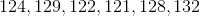Explanation:

To solve, first order the numbers from least to greatest: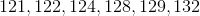Then, subtract the smallest number from the largest: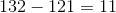Answer: The range is.

All ISEE Middle Level Math Resources